# Report Formula

Zoho Analytics provides an option named Report Formula. This enables you to perform specific calculations in the report using the metric in the report.

### 1. What is Report Formula?

Report formula is a formula column specific to a particular report. It differs from Formula Column and Aggregate Formula which are based over a table (which are then available for creating any report on top of the table).

Report Formula allows you to use the basic arithmetic operators such as +, -, * and / over the columns dropped in the Data shelf of the report. The report formula will be added as a metric in the report.

### 2. On what views can I create a Report Formula?

You can create Report Formula over the following report types:

• Chart
• Pivot Table
• Summary View

### 3. How do I create a Report Formula?

You can create a Report Formula from the report editor. Follow the below steps to create a Report Formula.

1. Open the report in Edit mode.
2. Click the icon.
For Charts, this will be available on the left of the data shelves. For Pivot Table and Summary View, this will be available on the right of the data shelves.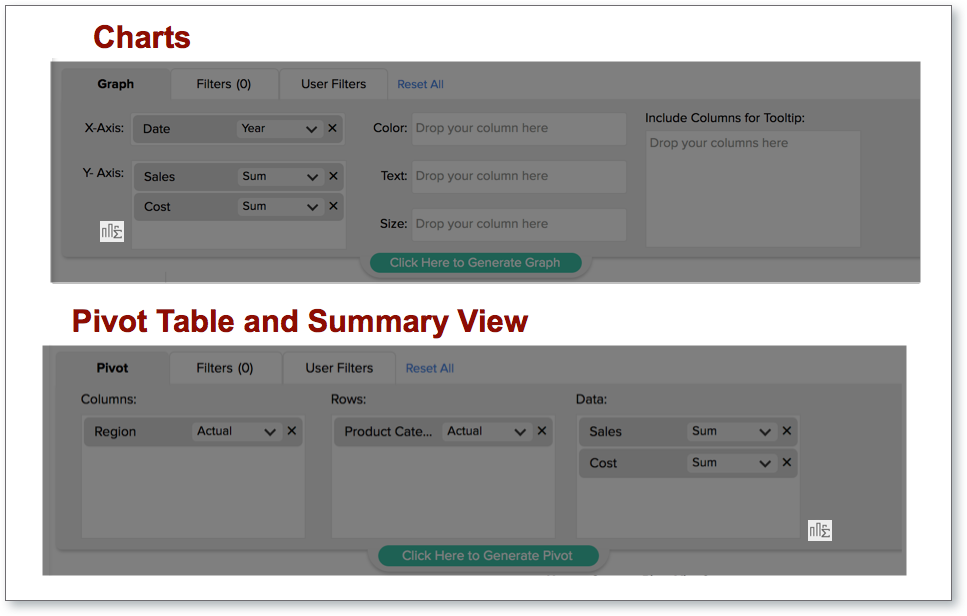3. The Add Formula dialog will open. Provide a name for your Formula.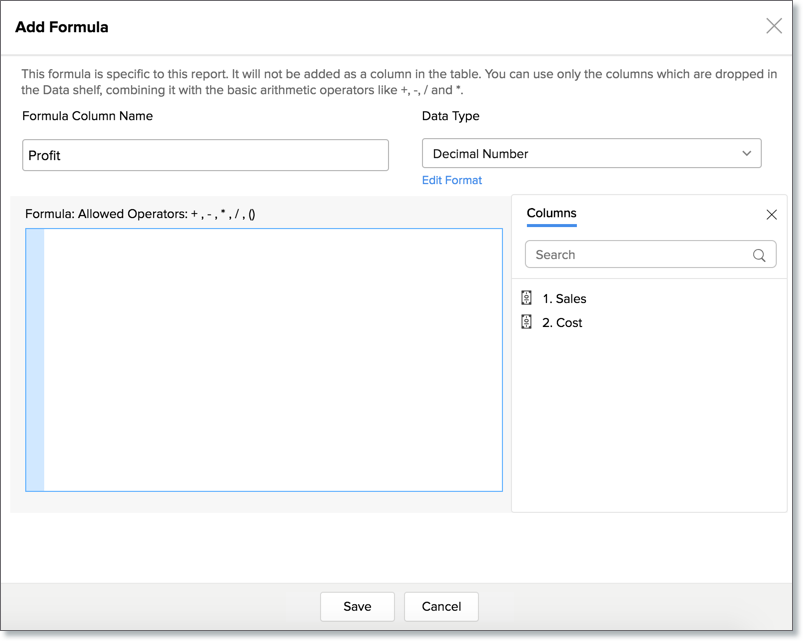4. Select the Data type of the column.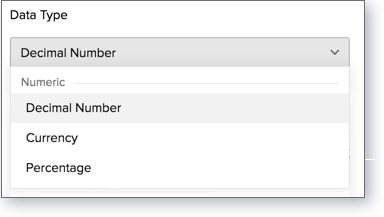5. Specify the formula in the editor. You can perform any calculations in the report using the metric in the report. Report formula supports IF condition, and the following arithmetic operators, logical operators, and comparison operators.
• Arithmetic Operators -  +, -, *, /, and ()
• Comparison Operators - >, <, >=, <=, =, and !=
• Logical Operators - and, or, and null
6. Click Save. The created Report Formula will be added as a metric in the report.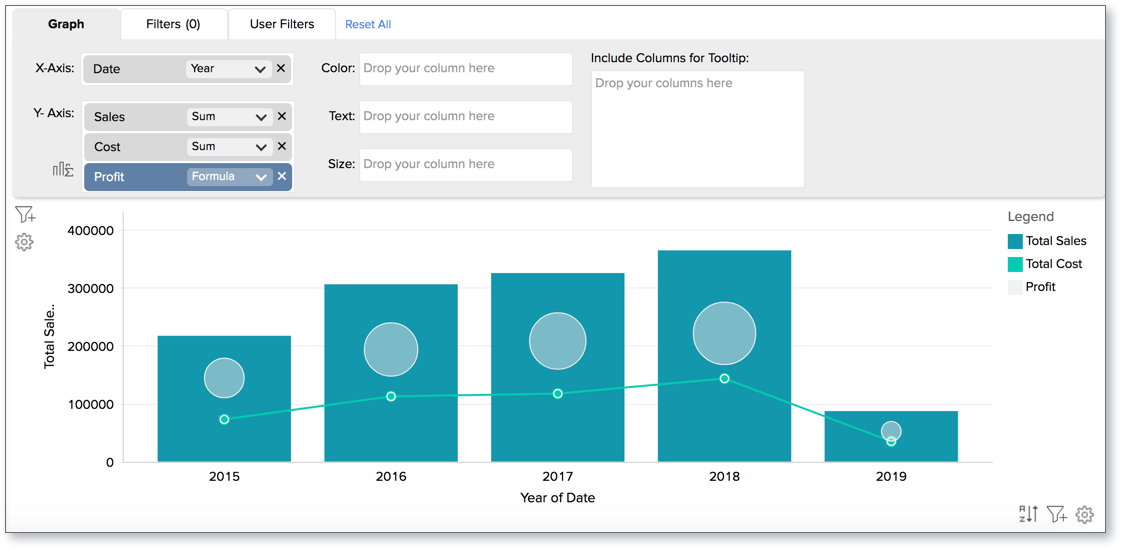Note: It will not be added as a column in the table.

The following presentation explains how to calculate the Magic Number. This is a widely used formula to measure sales growth.

### 4. How do I edit Report Formula?

You can edit a Report Formula by following the steps below.

1. Open the report in Edit mode.
2. Click the column in the Data shelf and then click Edit Formula.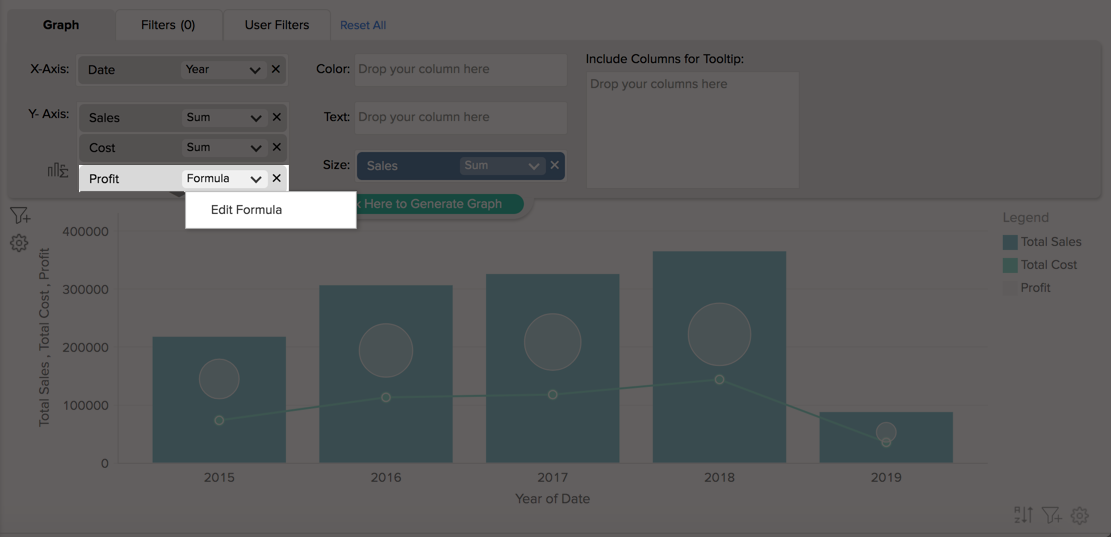3. The formula dialog will open. Edit the formula as required.

### 5. Can I use a Report Formula of one report in another report?

No, formulas created over a report are specific to that report alone, and cannot be used in other reports.